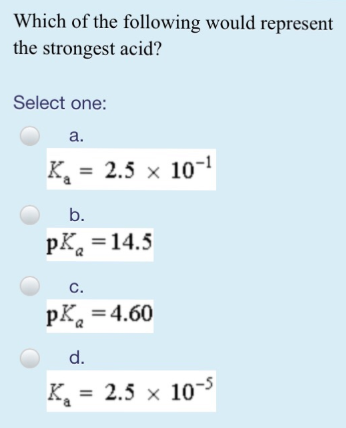# Problem: Which of the following would represent the strongest acid? Select one: a. Ka = 2.5 × 10-1 b. pKa = 14.5 c. pka = 4.60 d. Ka = 2.5 × 10-5

###### FREE Expert Solution
100% (399 ratings)###### Problem Details

Which of the following would represent the strongest acid?

Select one:

a. Ka = 2.5 × 10-1

b. pKa = 14.5

c. pka = 4.60

d. K= 2.5 × 10-5